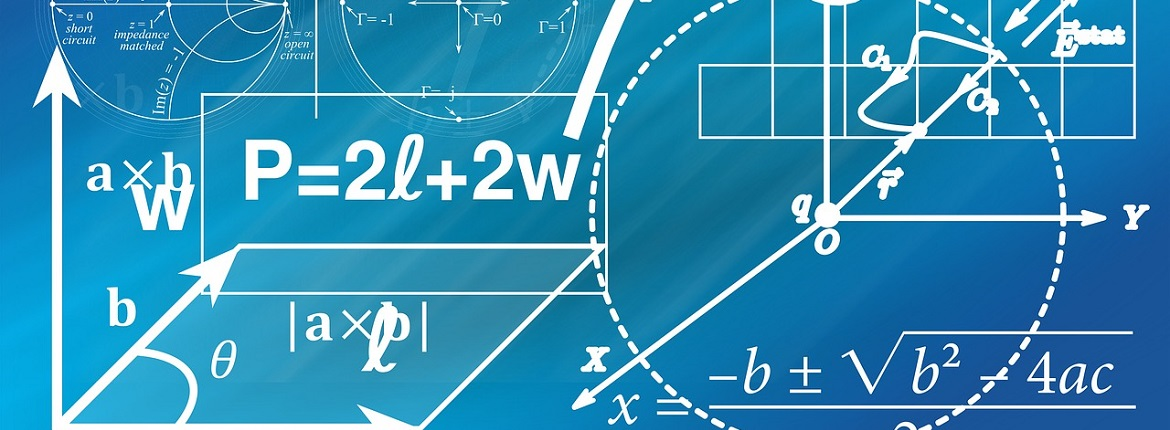## Visakha Government Degree College for Women

Accredited by NAAC with 'B' Grade

Affiliated to A.U

Bharat padhe online

LMS

 Latest News / Events Online Timetable for UG & PG     |     Bharath padhe online - This quarantine study from home

### Useful Links## About Mathematics Department

Mathematics (from Greek μάθημα máthēma, "knowledge, study, learning") includes the study of such topics as quantity (number theory), structure (algebra), space (geometry), and change (mathematical analysis). It has no generally accepted definition.

Mathematicians seek and use patterns to formulate new conjectures; they resolve the truth or falsity of conjectures by mathematical proof. When mathematical structures are good models of real phenomena, mathematical reasoning can be used to provide insight or predictions about nature. Through the use of abstraction and logic, mathematics developed from counting, calculation, measurement, and the systematic study of the shapes and motions of physical objects. Practical mathematics has been a human activity from as far back as written records exist. The research required to solve mathematical problems can take years or even centuries of sustained inquiry.

Mathematics is essential in many fields, including natural science, engineering, medicine, finance, and the social sciences. Applied mathematics has led to entirely new mathematical disciplines, such as statistics and game theory. Mathematicians engage in pure mathematics (mathematics for its own sake) without having any application in mind, but practical applications for what began as pure mathematics are often discovered later.

Mathematics arises from many different kinds of problems. At first these were found in commerce, land measurement, architecture and later astronomy; today, all sciences suggest problems studied by mathematicians, and many problems arise within mathematics itself. For example, the physicist Richard Feynman invented the path integral formulation of quantum mechanics using a combination of mathematical reasoning and physical insight, and today's string theory, a still-developing scientific theory which attempts to unify the four fundamental forces of nature, continues to inspire new mathematics

Some mathematics is relevant only in the area that inspired it, and is applied to solve further problems in that area. But often mathematics inspired by one area proves useful in many areas, and joins the general stock of mathematical concepts. A distinction is often made between pure mathematics and applied mathematics. However pure mathematics topics often turn out to have applications, e.g. number theory in cryptography. This remarkable fact, that even the "purest" mathematics often turns out to have practical applications, is what Eugene Wigner has called "the unreasonable effectiveness of mathematics".